# Statistics Tutorial 2 – Central Limit Theorem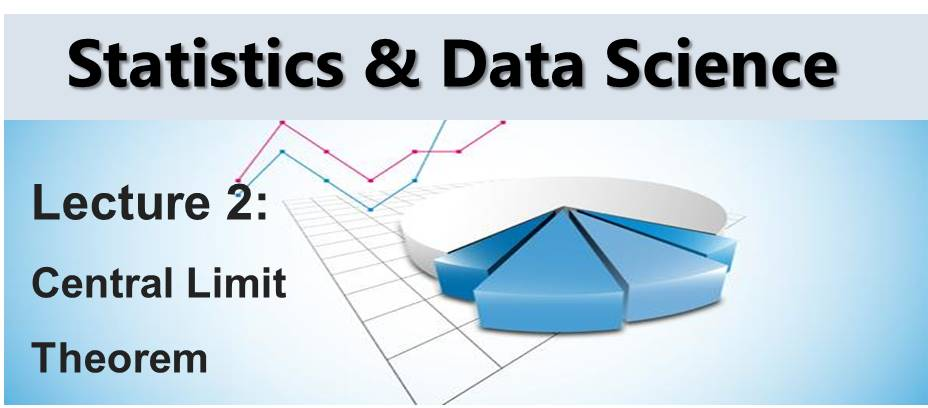In this tutorial, we would discuss the Central Limit Theorem.

What is Central Limit Theorem

This is a theorem (or set of theorems) that applies in both statistics and probability theory. It has it that if set of samples with sufficiently large size and finite level of variance are taken from a population, then the mean of all the samples will be approximately equal to the mean of the population.

Central Limit Theorem Example

So assume we have a population with a mean of µ and a standard deviation of σ.

Sample: Then we take samples  of size n from this population.

Let’s take a population with distribution shown in Figure 1. We take several samples from this population as tabulated in Table 1.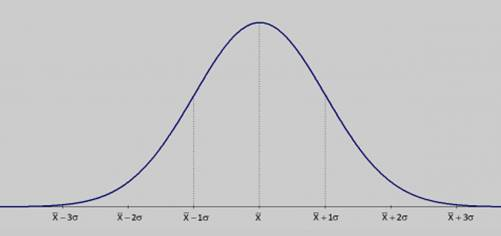Figure 1: Some Population

 Sample 1 Sample 2 Sample 3 value 1 value 1 value 1 value 2 value 2 value 2 Mean 1 Mean 2 Mean 3

Table 1: Samples from a population

Theorem a: The mean of the sample means equals the population mean. The formula is shown below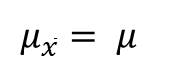Theorem b: The standard deviation of the sample means equal the population mean divided by square root of n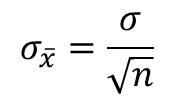Theorem c: If  the population is normal, then the sample mean will have a normal distribution. This is irrespective of the sample size.

Theorem d: If the population is not normal but the sample size n > 30, then the sampling distribution of the sample means approximates to a normal distribution.

Example 2

Given a population mean and standard deviation as below:

μ = 35

σ = 9

And a sample size n = 64

• Calculate the mean of the sampling distribution
• Also calculate the standard deviation of the sampling distribution

Solution

Using theorem d, the mean of the sample means = mean the population = 35.

Using theorem c, we can calculate the  standard deviation of the sample means as: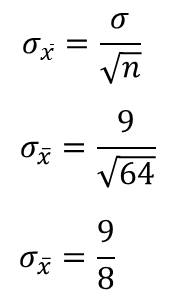Exercise on Applying the Central Limit Theorem

The mean IQ of a certain population is 100 with a standard deviation of  15. If a sample of 50 persons was taken, what is the probability that the average IQ of the sample is greater than 105?

I hope this is very clear. We would now proceed to learn about Theory of Estimation: